Elementary Algebra 2e

# Key Concepts

### Key Concepts

#### 5.1Solve Systems of Equations by Graphing

• To solve a system of linear equations by graphing
1. Step 1. Graph the first equation.
2. Step 2. Graph the second equation on the same rectangular coordinate system.
3. Step 3. Determine whether the lines intersect, are parallel, or are the same line.
4. Step 4. Identify the solution to the system.
If the lines intersect, identify the point of intersection. Check to make sure it is a solution to both equations. This is the solution to the system.
If the lines are parallel, the system has no solution.
If the lines are the same, the system has an infinite number of solutions.
5. Step 5. Check the solution in both equations.

• Determine the number of solutions from the graph of a linear system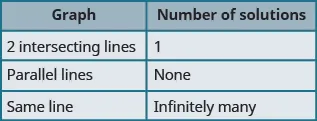• Determine the number of solutions of a linear system by looking at the slopes and intercepts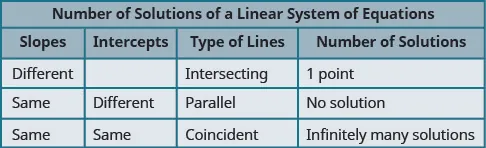• Determine the number of solutions and how to classify a system of equations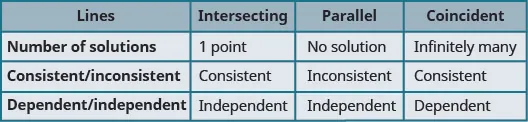• Problem Solving Strategy for Systems of Linear Equations
1. Step 1. Read the problem. Make sure all the words and ideas are understood.
2. Step 2. Identify what we are looking for.
3. Step 3. Name what we are looking for. Choose variables to represent those quantities.
4. Step 4. Translate into a system of equations.
5. Step 5. Solve the system of equations using good algebra techniques.
6. Step 6. Check the answer in the problem and make sure it makes sense.
7. Step 7. Answer the question with a complete sentence.

#### 5.2Solving Systems of Equations by Substitution

• Solve a system of equations by substitution
1. Step 1. Solve one of the equations for either variable.
2. Step 2. Substitute the expression from Step 1 into the other equation.
3. Step 3. Solve the resulting equation.
4. Step 4. Substitute the solution in Step 3 into one of the original equations to find the other variable.
5. Step 5. Write the solution as an ordered pair.
6. Step 6. Check that the ordered pair is a solution to both original equations.

#### 5.3Solve Systems of Equations by Elimination

• To Solve a System of Equations by Elimination
1. Step 1. Write both equations in standard form. If any coefficients are fractions, clear them.
2. Step 2.
Make the coefficients of one variable opposites.
• Decide which variable you will eliminate.
• Multiply one or both equations so that the coefficients of that variable are opposites.
3. Step 3. Add the equations resulting from Step 2 to eliminate one variable.
4. Step 4. Solve for the remaining variable.
5. Step 5. Substitute the solution from Step 4 into one of the original equations. Then solve for the other variable.
6. Step 6. Write the solution as an ordered pair.
7. Step 7. Check that the ordered pair is a solution to both original equations.

#### 5.5Solve Mixture Applications with Systems of Equations

• Table for coin and mixture applications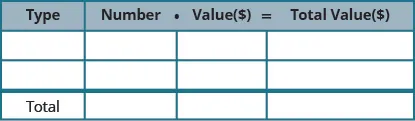• Table for concentration applications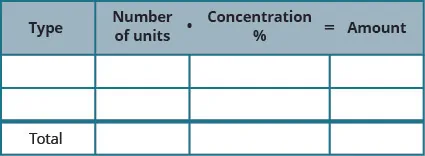• Table for interest applications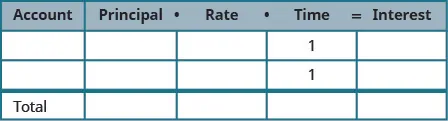#### 5.6Graphing Systems of Linear Inequalities

• To Solve a System of Linear Inequalities by Graphing
1. Step 1.
Graph the first inequality.
• Graph the boundary line.
• Shade in the side of the boundary line where the inequality is true.
2. Step 2.
On the same grid, graph the second inequality.
• Graph the boundary line.
• Shade in the side of that boundary line where the inequality is true.
3. Step 3. The solution is the region where the shading overlaps.
4. Step 4. Check by choosing a test point.
Order a print copy

As an Amazon Associate we earn from qualifying purchases.